Hydrostatic Force

# Hydrostatic Force | Mechanical Engineering SSC JE (Technical)

 1 Crore+ students have signed up on EduRev. Have you?

HYDROSTATIC FORCE

• Pressure Force on Plane Surfaces 
• Pressure force always acts normal to plane surface. 
• The center of pressure passes through centroid of the pressure diagram. 
• In horizontal plane surface pressure force passes through centroid of area whereas in inclined and vertical plane surfaces pressure force passes below the centroid of the area. 
• The total pressure force on plane area is
F = Area × Pressure at the centroid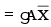where,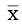= vertical depth of centroid of area from free liquid surface A = Area of surface 
• Depth of center of pressure from free surface is given by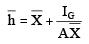where, IG = Moment of inertia about centre of gravity of the body. 

• Center of pressure always lies below the centroid of the area in non-horizontal surfaces. 
• The depth of center of pressure on inclined plane is given by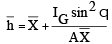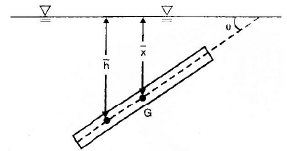• Pressure Force on curved surfaces: Horizontal component of the resultant hydrostatic force 'Fx' of curved surface may be computed by projecting the surface upon a vertical plane and multiplying the projected area by the pressure at its own centre of area. 
• Vertical component of force 'Fy' is equal to the weight of the liquid block lying above the curved surface upto free surface.
• Resultant Force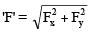• Angle of line of action of resultant force with the horizontal is given by tan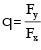• Depth of center of pressure for some vertical plane surfaces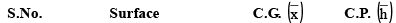1. RECTANGLE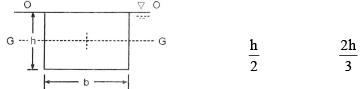2. TRAPEZIUM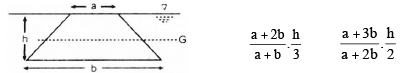3. TRIANGLE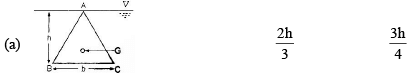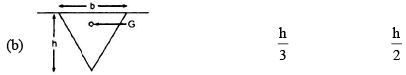4. CIRCLE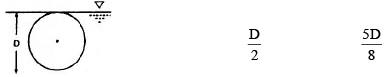5. SEMI CIRCLE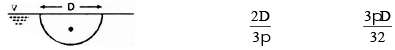6. PARABOLA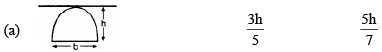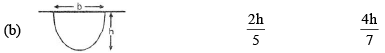• Archimedes principle and Force of buoyancy
• When a body is submerged either fully or partially then it is acted upon by a force of buoyancy vertically up ward which is equal to weight of liquid displaced by the body. This force of buoyancy always acts through the centroid of liquid displaced. 
• Stability of submerged bodies
If centre of buoyancy (B) and centre of gravity (G), coincide then submerged body is in neutral equilibrium & if  B is above G it will be in stable equilibrium.
If B is below G it will be in unstable equilibrium.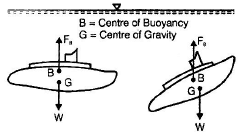• Stability of floating bodies: The stability of floating body is defined by relative position of metacentre (M) and centre of gravity (G).
If M lies above G (GM ve) then it is stable equilibrium
If M lies below G (GM  - ve) then unstable equilibrium
If M coincides with G (GM  0) then neutral equilibrium
é =+ù êú êú ê =ú êú êú êë =úû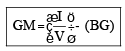where GM = metacentric height.
I = moment of inertia about longitudinal axis of the plane at the level of free surface. ('I' is minimum moment of inertial of the plane.) V = Volume of liquid displaced by the body.
BG = Distance between centroid of liquid displaced and centroid of the body.
• For stable equilibrium of a circular cone floating with its apex downward having diameter 'd' and a vertical height 'h'. (The specific gravity of the cone is 'S') the condition for stability is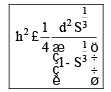Depth of submergence is x = S1/3h
• If a floating body oscillates then its time period of transverse oscillation is given by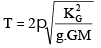• Vortex Motion :
A whirling mass of fluid is called vortex flow.
(i) Forced Vortex Flow: 
• When a fluid is rotated about a vertical axis at constant speed, such every particle of its has the same angular velocity, motion is known as the forced vortex. Forced vortex requires constant supply of external energy/torque.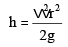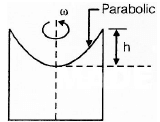where h is height of paraboloid, and r is radius of paraboloid at top.
Volume of paraboloid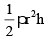= ½ of volume of circumscribing cylinder.

• The surface profile of forced vortex flow is parabolic.
Example of forced vortex flow is rotating cylinder & flow inside centrifugal pump.

(ii) Free Vortex Flow: 
• In this flow fluid mass rotates due to conservation of angular momentum. No external torque or energy is required. In free vortex flow Bernoulli's equation can be applied.  The velocity profile is inversely proportional with the radius.
v r = constant
The point at the centre of rotation is called singular point, where velocity approaches to infinite.
Example of free vortex motion are whirling mass of liquid in wash basin, whirlpool in rivers etc.
The document Hydrostatic Force | Mechanical Engineering SSC JE (Technical) is a part of the Mechanical Engineering Course Mechanical Engineering SSC JE (Technical).
All you need of Mechanical Engineering at this link: Mechanical Engineering

## Mechanical Engineering SSC JE (Technical)

6 videos|97 docs|57 tests

## Mechanical Engineering SSC JE (Technical)

6 videos|97 docs|57 tests

Track your progress, build streaks, highlight & save important lessons and more!(Scan QR code)

,

,

,

,

,

,

,

,

,

,

,

,

,

,

,

,

,

,

,

,

,

;